# Using F.A.C.E. to Convert Raw Data Units

We here at FlowWorks are often asked whether raw data can be converted and stored in different units, than that of which is originally provided by the data logger. The short answer is yes - but it must be done without modifying the original data.

Retaining all data in the same units as provided by the data logger is a best practice we maintain – if an analysis or conversion of the data were to go wrong, there is always the raw, ‘unaltered’ data upon which to fall back. For this reason, we do not support any alterations to the data from within the original channel that it came.

Instead, we recommend using FlowWorks' Advanced Calculation Engine (FACE) to convert data. Using FACE, users can set up a new calculated channel that consistently copies-in data from the original channel and applies a desired unit conversion formula. The resultant data in the calculated channel can be used for any form of analysis in lieu of the original channel, thus leaving the original channel untouched in case a back-up is ever needed.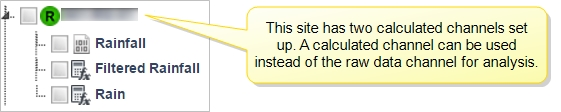## Setting up a new calculated channel for data conversion

The process of setting-up a new calculated channel is two-fold: first, you need to create a new channel; second, you need to configure the channel to perform the calculation.

To set up a new calculated channel:

1. Log-in to your FlowWorks user account.
2. Hover your mouse over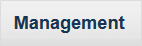, found in the Main Toolbar.
3. Select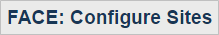, to open the Configure Sites page.
4. Click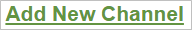. You will be directed to the Add channel page, where you will create the calculated channel.
5. Choose Site for the calculated channel to reside in. Typically, this should be the same site that contains the original channel.
6. Enter a Channel Name for the calculated channel - for easy reference, we recommend including the name of the original channel.
7. Choose the Channel Units.
8. For Channel Type, select 'Calculated' - remember, we want to apply a calculation to the raw data in order to convert its units of measure.
9. Ensure Visible is checked, to enable use of the calculated channel in management tools (such as Graphing and Reporting); check System Admin Only if you want to restrict visibility of the calculated channel just to system administrators.
10. Select Enable Rainfall Tools if your new channel is to collect rainfall-related data. Doing so will enable the channel's use in many of FlowWorks' rainfall analysis tools, such as the I&I Estimator and Rainfall IDF Analysis.
11. Optionally, add the new calculated channel to other sites. Expand Advanced Options, and select the desired sites.
Note: The Site list in Advanced Options contains only those sites that do not already have a channel with the same name.
1. Click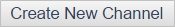. The system may need a few moments to process - you will be directed back to the 'Configure Sites' page once complete.Now that a new calculated channel has been created, you need to configure it to perform the conversion calculation.

## Configuring a Calculated Channel using a Formula

Formula is one of many calculation types you can choose from; it is a popular one because it enables you to manually create any type of equation using basic mathematical notation.

1. From the 'Configure Sites' page, select the calculated channel. Expand the Choose Sites list and select the site that your new calculated channel resides in; then, from the Choose Calculated Channel list, select the new channel you created in the previous section.
Note: No action is required for the Choose Channel menu.
1. Click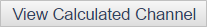.
2. Click.
3. Give the calculation a name and description (optional).
4. Select the calculation Type. There are a number of options to choose from - including Sum and Rolling Average. Often, Formula is chosen because it allows you to manually input a mathematical equation, that is to be applied to all incoming raw data. Upon choosing an option, the bottom section of the screen will update, revealing further questions that must be answered to set up the calculation. If you have chosen Formula, you can define the equation - the remainder of this section explains how to use the Formula option.
5. Set a Start Date and End Date (optional).
6. Define the Source Components. For each component, select the source site and channel and assign it a variable name (any letter). Use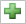to add more.
7. Choose a Lookup Table (optional). The lookup function allows the use of a lookup table in a formula. Choose the lookup table from the drop-down list and reference in the formula with an input channel, such as LOOKUP (A).
8. Define the equation - in the Define Equation box, use basic math syntax to express the conversion formula. Some due diligence may be needed to ensure the formula outputs the correct conversion.
9. Expand the Advanced Options drop-down, and select all options that apply. You can also add this calculation to other sites.
10. Click. You will be directed back to the 'View Calculated Channel' page, where the new calculation will appear in a list.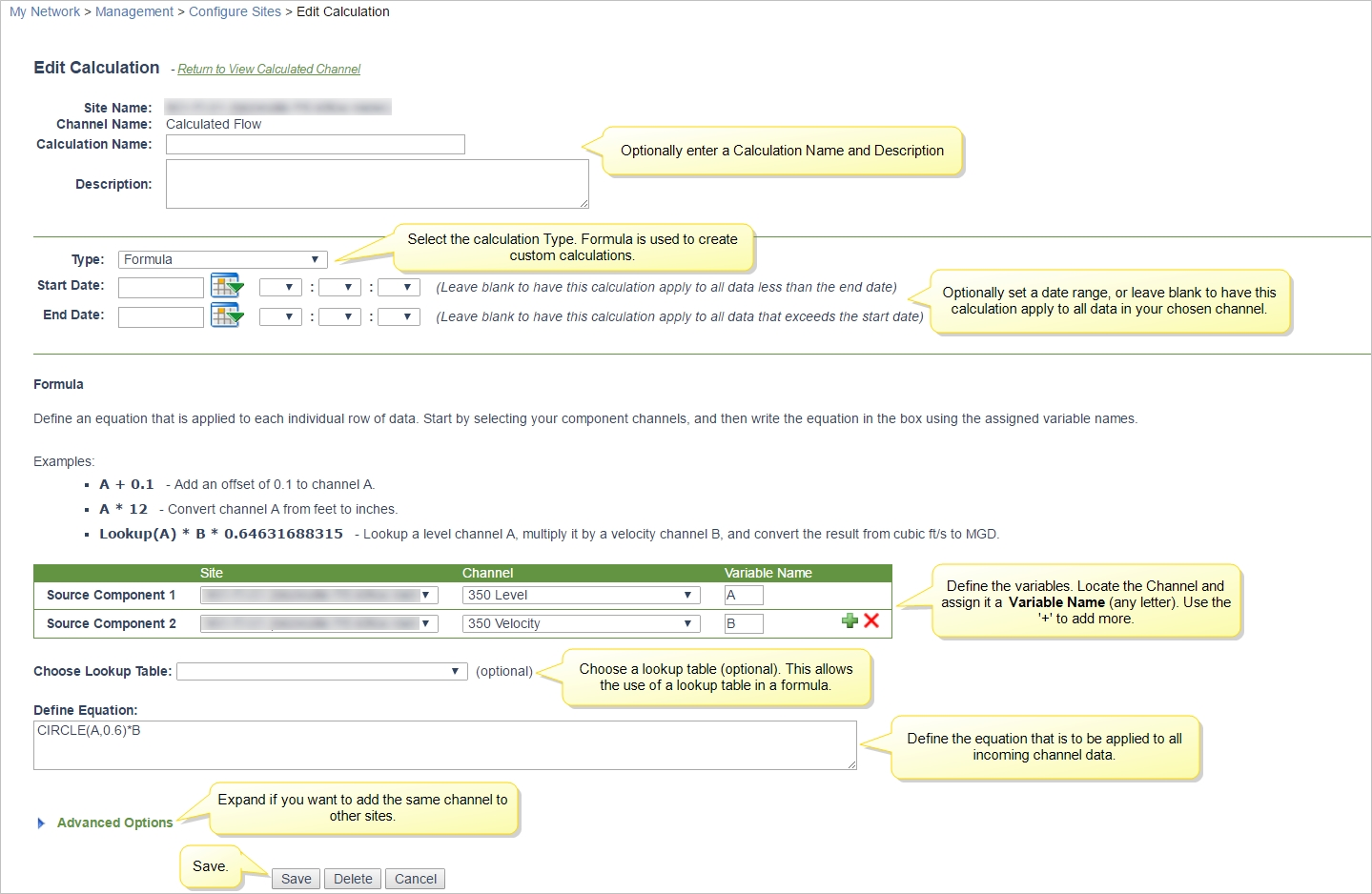Your new calculated channel is now set up and ready for use. All incoming data will be copied into the calculated channel from the source channel, where the conversion formula will be automatically applied. You can use this channel in lieu of the original channel with any of the available analysis and management tools.

# Have questions?

Feel free to reach our support team by submitting a new support ticket.